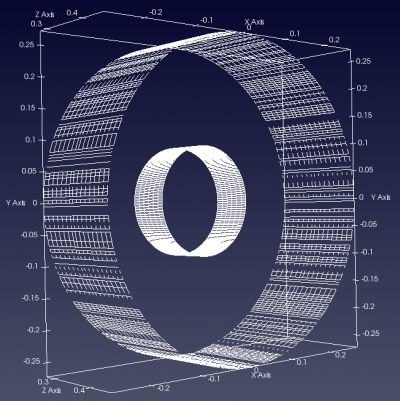# Box

The Box is the most simple primitive in CSXCAD. It is as well the most used primitive since it usually matches the given Cartesian or cylindrical FDTD mesh. Furthermore this primitive is the only one which shape depends on the chosen coordinate system it is defined with.

## Description

The box primitive is defined by two coordinates, e.g. start and stop:

```CSX = AddBox(CSX, 'propName', 1, start, stop, varargin);
```

with the following parameters:

• CSX: The original CSX structure
• propName: name of the assigned property
• prio: priority of the primitive
• start: [x y z] first/start coordinate
• stop: [x y z] second/stop coordinate
• varargin: a key/value list of primitives variable arguments

## Examples

• Create a Cartesian box from x=[-100 to +100], y=[-50 to 0] and z=[-50 to 10]
```CSX = AddBox(CSX,'metal',1,[-100 -50 10],[100 0 -50]);
```
• In case of a cylindrical system, create a cylindrical box from r=[50 to 70], alpha=[pi/2 to 3*pi/2] and z=[-50 to 10]:
```CSX = AddBox(CSX,'metal',1,[50 pi/2 10],[70 3*pi/2 -50]);
```

Note that although AddCylindricalShell may appear to be the appropriate function to define a cylinder when using a cylindrical coordinate system, AddBox is actually better suited because the structure will be meshed correctly. If AddCylindricalShell is used, it is possible that the meshed cylinder will not be meshed correctly, as shown in the example below.

• In case of a Cartesian FDTD setup, define a cylindrically shaped box from r=[50 to 70], alpha=[pi/2 to 3*pi/2] and z=[-50 to 10]:
```CSX = AddBox(CSX,'metal',1,[50 pi/2 10],[70 3*pi/2 -50], 'CoordSystem', 1);
```Comparison of cylinders defined with AddCylindricalShell (outer) and AddBox (inner).